﻿ Modeling and Analysis of Cholera Dynamics with VaccinationPublications are Open
Access in this journal
Article Versions
Export Article
• Normal Style
• MLA Style
• APA Style
• Chicago Style
Research Article
Open Access Peer-reviewed

### Modeling and Analysis of Cholera Dynamics with Vaccination

Nneamaka Judith Ezeagu , Houénafa Alain Togbenon, Edwin Moyo
American Journal of Applied Mathematics and Statistics. 2019, 7(1), 1-8. DOI: 10.12691/ajams-7-1-1
Received November 12, 2018; Revised December 14, 2018; Accepted December 24, 2018

### Abstract

A mathematical model for the transmission of cholera dynamics with a class of quarantined and vaccination parameter as control strategies is proposed in this paper. It is shown through mathematical analysis that the solution of the model uniquely exist, is positive and bounded in a certain region. The disease-free and endemic equilibrium points of the model are obtained. By using the next generation matrix, the basic reproduction number was computed around the disease-free equilibrium points, and it was shown through the Jacobian matrix that the disease free equilibrium is locally asymptotic stable if Rh<1. Numerical simulation was carried to understand the impact of the incorporated controls as the system evolves over time. Results show that effective quarantine, vaccination and proper sanitation reduce the disease contact rates and thus eliminates the spread of cholera.

### 1. Introduction

The world health organization defines cholera as an acute diarrheal disease of the intestine caused by the bacterium Vibrio cholerae 1. Cholera is contracted through ingestion of food or water contaminated with Vibrio cholerae, and the symptoms includes profuse watery diarrhea, vomiting, and extreme dehydration in severe cases 2. It is also noted that most infected people are asymptomatic but the bacteria are present in their stool for some days after infection. These bacteria are shed back into the environment which potentially infect other people. According to a study by Azman et al. 2, cholera infected persons takes 0.5 days to 4.4 days to show symptoms. It is also reported that cholera can kill within hours if left untreated. On estimation, cholera causes roughly 1.3 to 4 millions cases and 21000 to 143000 deaths annually 1. The number of cholera reported cases has continued to be high over the last few years 3 with countries like Zimbabwe, Vietnam, Nigeria, Haiti 4, 5, and Zambia experiencing the most recent cholera outbreak. According to the government statement of Tuesday 23rd January 2018, the recent cholera outbreak in Zambia have killed 78 of the more than 3,600 people who had fallen sick since October, 2017. The sources of infection transmission in cholera outbreaks is associated with inadequate access to sanitation facilities and clean water. Therefore, it is important to note the different possible cholera transmission pathways are human to human and environment to human. High risk areas potential of having Vibrio cholerae include overcrowded camps for refugees, and slums where there is less or no access to clean water and sanitation systems. Although cholera may be life threatening but controlling and preventing the disease is normally straight forward if proper sanitation practices are followed 6. Also, a key to control cholera and to reduce deaths is by employing a multifaceted approach. Therefore, a combination of proper sanitation and hygiene, surveillance, education awareness, oral rehydration solution (ORS), cholera vaccines are used 1.

A good understanding of the transmission dynamics of cholera is crucial for effective control, prevention and intervention strategies against cholera outbreak. Through mathematical modeling, understanding into the transmission dynamics of cholera is gained and consequently helps for prediction. Several studies have been elicited in the quest to gain deeper understanding of the complex dynamics of cholera. Mathematical models have been used in several studies as a tool that can provide a quantitative and potentially valuable outcome in the fight against cholera. We give a review of a few related works while we recommend for more references be found from the referenced papers. Codeço 7 proposed an SI-B (Susceptible, Infected and Bacteria) cholera model to analyze the effect of aquatic reservoir in the persistence of endemic cholera. The author assumed the rate individuals are born is the same as the rate they die. Ochoche 6 modified Codeço’s model with addition of control strategy and different birth and death rate. Because of the large impact of vaccination in controlling diseases, Cui et al. 8 developed an SIVR-B model with the variable, V as the class of vaccinated individuals, and R, the recovered class. Building on the model in 6, Mukandavire et al. 9 proposed an SIR-B model for the 2008-2009 outbreak in Zimbabwe which considers a fast and slow environmental transmission. The slow transmission in this sense is said to be an environment-to-human transmission characterized with a high infectious dose. It results from drinking from water bodies contaminated with V. cholerae which have been in the environment for a long period (5 to 18 h) to no longer be hyperinfectious. Whereas, the fast transmission is assumed to be that of human-to-human characterized with a low infectious dose which results from consuming water or food contaminated with hyperinfectious vibrios from freshly passed human stool. Wang and Modnak 10 extended the model in 9 capturing the effects of therapeutic treatment, vaccination, and water sanitation. The optimal control strategy was analyzed in other to obtain the best cost-effective multiple time-dependent intervention strategies. Edward and Nyerere 5 modified the model in 9 to include public health educational campaigns parameter in addition to the control strategies in 10. Lemos-Paião et al. 11 proposed a model with the class of individual under treatment through quarantine (Q). It is also assumed the recovered individuals can lose immunity and become susceptible again. In order to obtain a successful treatment, the authors analyzed the optimal cost-effective quarantine strategy for the minimization of the number of infectious individuals and bacteria concentration. Sun et al. 12 incorporated vaccination and disinfection as the basic control strategies in a mathematical study on the SIR-B model of cholera transmission dynamics. The authors took into account the two transmission paths considered in Mukandavire et al. 9.

Motivated by the work in 11, we therefore aim to modify the model proposed in the paper with the addition of the assumption that once an individual is vaccinated or treated, they do not loose immunity. Though, research reports that cholera vaccines do not offer complete protection 13. However, as the global strategy on cholera control aims to reduce cholera deaths and eliminate cholera 1, we therefore study to understand the scenario where recovered individuals do not have to lose immunity in the same cholera outbreak.

The rest of the paper is organized as follows. In Section 2, we describe and formulate the model for the dynamics of cholera transmission and give the description of the model parameters and variables in Table 1. Through mathematical analysis, we analyze the model properties in Section 3. In Section 4, we present the numerical results in different graphs and give the result discussion. Finally, we give the conclusion in Section 5.

### 2. Model Assumptions and Formulation

We propose an SIQR epidemic model with a class of bacterial concentration for the dynamics of cholera. Our model is motivated by the work of Lemos-Paião et al. 11, and we incorporate the two transmission paths considered in 9. The total human population, N(t) at time t ≥ 0 is assumed to be non constant and divided into four classes: susceptible, S(t), symptomatically infectious, I(t), quarantine isolated, Q(t) and the recovered class, R(t). Individuals are recruited into the susceptible class at an average rate, Π and die naturally at a rate, μ. The vibrios concentration in contaminated water is denoted by B(t). Cholera infection is contracted by the susceptible individuals either by ingesting vibrios from contaminated aquatic reservoirs at a rate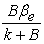or through human-to-human transmission which results from the ingestion of hyperinfectious vibrios 9 at a rate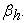Note that the subscripts, e and h respectively denotes environment-to-human and human-to-human. We denote by k, the concentration of V. cholerae in the environment that yields 50% chances of contracting cholera, and,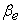and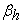are respectively the rates of ingesting vibrios from the contaminated environment and through human-to-human interaction. environment and through human-to-human interaction. The susceptible individuals move to the recovery class after being vaccinated at a rate,and never loose their immunity in the same cholera outbreak. Infected and quarantined individuals die as a result of cholera disease at the rates,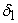and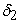respectively. Through shedding, infectious individuals contribute to V. cholerae in the environment at a rate,. Furthermore, the vibrios die at a rate, c after proper environmental sanitation. Once individuals are observed to have been infected, they are isolated through hospital quarantine at a rate,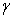. Upon successful therapeutic treatment, the quarantined individual progress to the recovery class at a rate. These assumptions are therefore translated into the following nonlinear differential equations:(1)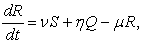with the nonnegative initial conditions:The model parameters and variables are described with values in Table 1 and note that the human population,### 3. Mathematical Analysis

3.1. Basic Properties of the Model

The model system describes the dynamic of human population and V.cholerae in the aquatic reservoir. Before we embark on analyzing the dynamics of the model, we first discus the basic properties of the system. All model variables and parameters are assumed to be nonnegative for all t ≥ 0 since the model monitors changes in the population.

3.1.1. Existence of Solution

Letand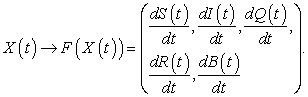Then the system (1) becomes,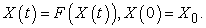Theorem 1. (Existence and Uniqueness) The model (1) is continuous and satisfies the Cauchy-Lipschitz condition.

Proof. We show for one equation and the rest follows the same procedure. Let(3)

Then,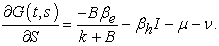(4)

We have that the function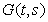and its partial derivative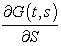are defined and continuous at all points (t, S). Similarly, the right-hand functions of the other equations and their respective partial derivatives satisfy these conditions. Hence by the existence, and uniqueness theorems, there exists a unique solution for S(t), I(t), Q(t) and R(t) in some open intervals centered at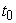. We move on to show that the solution satisfies the Lipschitz condition. Using equation (3), we see thatThis implies that, whereis a Lipschitz constant. In a similar way, we obtained that the remaining variables satisfy the Lipschitz condition and thus, there exists a unique solution S(t), I(t), Q(t), B(t) for all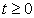.

Theorem 2. (Positivity) Given the non-negative initial conditions (2), then the solutions S(t), I(t), Q(t), R(t) and B(t) are non-negative for all.

Proof. From the first equation in model (1), we deduce that for all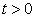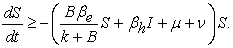(5)

Letthen equation (5) becomes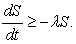(6)

Integrating equation (6) givesHence,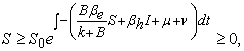(7)

whereis the susceptible population at t=0. Similarly, we obtain from the last four equations of model (1) that I(t), R(t), Q(t) and B(t)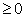. Therefore, any solution of model of model (1) is such thatWe show in the following Theorem 3 that it is sufficient to consider the flow dynamics of model (1)-(2) in a certain region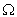.

Theorem 3. (Boundedness) All solution of model (1) is bounded and remain in the region.(8)

where(9)

and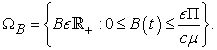(10)

Proof. We begin by splitting the model (1) into human population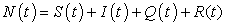and the bacteria population, B(t). Then we have that,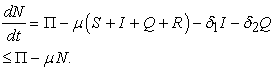By integrating, we obtainwhere C is a constant. Initially at t=0,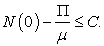Therefore,Thus,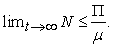(11)

Similarly,and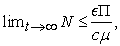(12)

This shows that human and bacteria population are biologically feasible in the region (9) and (10) respectively. Therefore, the solution of model (1) with the initial conditions (2) is bounded in the invariant region (8) for all. Hence, the model is well posed.

3.2. Equilibria Points and Stability Analysis

At each of the equilibrium points of the model (1),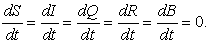Thus, we compute the following.

3.2.1. Disease Free Equilibrium

At the disease-free state, there is no disease in the human population and no bacteria in the environment which implies. Thus, the disease-free equilibrium of the model (1) is given by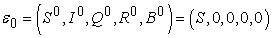(13)

with3.2.2. Endemic Equilibrium.

Let any arbitrary endemic equilibrium of the model system (1) be represented by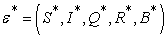such that. By solving the system, we obtain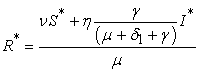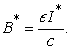3.2.3. Reproduction Number.

The basic reproduction number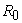as defined by Diekmann et al. 16 is the expected number of secondary cases produced, in a completely susceptible population, by a typical infected individual during its entire period of infectiousness. Using the method of next generation matrix explained in details by Van den Driessche and Watmough 17 we computefor the cholera model given in system (1) as follows: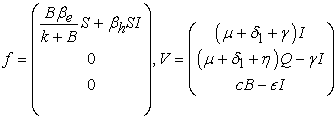where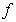is the rate of appearance of new infection,and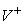and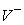respectively the rate of transfer of individual into the class by any other means and out of the compartment given by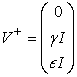and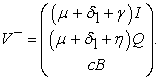Computing the derivatives ofand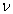with respect toat the DFE point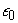, we obtain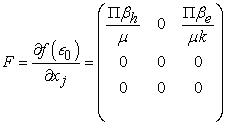and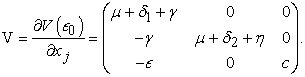Thus,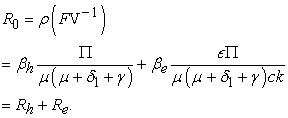In this case,represents the strands for the continuation of infectious individuals from the human to human interaction anddenotes the strand from the environment to human interaction. The epidemiological significance ofshows Cholera pandemic can effectively be controlled by reducing the contacts of infected individuals with other individuals that may include house members and curbing the environmental transmission of the disease. By so doing,will be brought to a value less than unity which implies that the disease can be eliminated from the population. This thus leads to the following theorem.

Theorem 4. The DFE ε0 of the model system (1) is locally asymptotically stable if Rh < 1, and unstable if Rh > 1.

Proof. Let J be the Jacobian matrix of the model system (1). Then, J at the disease free equilibrium point,is given as follows:The eigenvalues of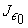are,,and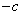. Clearly, all the eigen values except the first,are negative. Therefore,Thus, the disease-free equilibrium point is locally asymptotically stable if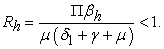### 4. Numerical Results and Discussion

In this section, we present a few graphs to illustrate the evolution of each population class over time. With special interest, we focus on the class of infected, quarantined, recovery and bacteria. Our focus here is based on the initial assumptions and therefore, we seek to assess the effect the control strategies have on the cholera transmission dynamics. Note that the graphs were obtained through numerical simulation of model (1) with the parameter values in Table 1. The MatLab computational software as used in 18, 19, 20 was used for the numerical simulation to obtain the time dependent solution of the model system.

It is observed in Figure 1 that there is a sharp increase in the population of the infected individual at the very early time. This means that even though interventions are incorporated, the infected individuals are not cured immediately. As the system evolves with time, the infected individuals begin to reduce until there is no infected person in the population.

However, the population of the quarantined individuals as shown in Figure 2 increases though at a very short time space just around the time a drastic decrease is observed in Figure 1. That is as soon as the infected population started reducing, the quarantined gradually increases in number. These explains the positive effect of isolating the infected individuals through quarantine to reduce the infection contact rates. Through early detection of infected persons and proper quarantining, the possibility of reducing and eliminating the infection becomes high.

Figure 3 shows the population of the recovered persons increases over time and then remains constant for a long time. It can be seen that the increase in R(t) starts about the time the population of Q(t) shown in Figure 2 starts decreasing. The change in R(t) results from the individuals who recovers from being quarantined as a result of the treatment placed on them. Also, by vaccinating the susceptible individuals, the immuned recovered population increases.

Figure 4 shows the growth curve of the bacteria populations. The graph shows that the bacteria population slightly increases then reduces exponentially. The exponential decrease implies that the intervention measures incorporated in the model was effective in eliminating the bacteria.

• Figure 1. Time evolution of infected individuals, I(t)
• Figure 2. Time evolution of the quarantined individuals, Q(t)
• Figure 3. Time evolution of the recovered individuals, R(t)
• Figure 4. Time evolution of the bacteria population, B(t)

### 5. Conclusion

In this paper, an SIQR-B mathematical model for the transmission dynamics of cholera diseases was formulated to a set of ordinary differential equations. The two possible transmission pathways: human-to-human and environment-to-human transmissions were considered. It was assumed that through vaccination, and proper therapeutic treatment while in quarantine, individuals recover and never lose immunity in same short cholera outbreak. Through mathematical analysis, the model solution was shown to uniquely exist, nonnegative and bounded in a certain region. To understand the stability conditions, the disease free equilibrium point was computed, and further, the expressions for the endemic equilibrium point were obtained. Further, the basic reproduction number was computed around the disease-free equilibrium points, and it was shown that the disease free equilibrium is locally asymptotic stable if Rh < 1. To understand the time evolution of the individual classes and the effect of vaccination, numerical simulation was done to obtain the time-dependent solution. It is deduced from the numerical results that by quarantining the infected individuals, the disease contact rate between the susceptible and infected is minimized which therefore reduces the spread of cholera in the population. For effective quarantine in order to minimize disease contact rate, there is need for surveillance for early detection of cholera cases. Also, education campaign and sensitization should be considered as good measures to help bring the contribution of environment-to-human transmission to minimal. Though, there is not yet a 100% proven effective cholera vaccine but results shows that through effective vaccination, there will cease to exist an infected individual as time evolves. Which implies that the disease will be eliminated in the community. Regular proper sanitation is necessary in order to have a Vibrio. cholerae free environment.

### References

  World Health Organization (WHO), “Cholera key facts,” 2018. [Online] Available: http://www.who.int/news-room/fact-sheets/detail/cholera. [Accessed Oct. 9, 2018] In article View Article  Azman, A.S., Rudolph, K.E., Cummings, D.A. and Lessler, J. “The incubation period of cholera: a systematic review,” Journal of Infection, 66(5), 432-438, 2013. In article View Article  PubMed  Yang, X., Chen, L. and Chen, J., “Permanence and positive periodic solution for the single-species nonautonomous delay diffusive models,” Computers & Mathematics with Applications, 32(4), 109-116, 1996. In article View Article  Chirambo, R., Mufunda, J., Songolo, P., Kachimba, J. and Vwalika, B., “Epidemiology of the 2016 cholera outbreak of chibombo district central zambia,” Medical Journal of Zambia, 43(2), 61-63, 2016. In article  Edward, S. and Nyerere, N. “A mathematical model for the dynamics of cholera with control measures,” Applied and Computational Mathematics, 4(2), 53-63, 2015. In article View Article  Ochoche, J.M., “A mathematical model for the transmission dynamics of cholera with control strategy,” International Journal of Science and Technology, 2(11), 797-803, 2013. In article  Codeco, C.T., “Endemic and epidemic dynamics of cholera: the role of the aquatic reservoir,” BMC Infectious diseases, 1(1), 1, 2001. In article View Article  PubMed  Cui, J., Wu, Z. and Zhou, X., “Mathematical analysis of a cholera model with vaccination,” Journal of Applied Mathematics, 2014. In article View Article  Mukandavire, Z., Liao, S., Wang, J., Gaff, H., Smith, D.L. and Morris, J.G., “Estimating the reproductive numbers for the 2008-2009 cholera outbreaks in zimbabwe,” Proceedings of the National Academy of Sciences, 108(21), 8767-8772, 2011. In article View Article  PubMed  Wang, J. and Modnak, C., “Modeling cholera dynamics with controls,” Canadian applied mathematics quarterly, 19(3), 255-273, 2011. In article  Lemos-Paião, A.P., Silva, C.J. and Torres, D.F., “An epidemic model for cholera with optimal control treatment,” Journal of Computational and Applied Mathematics, 318, 168-180, 2017. In article View Article  Sun, G.-Q., J.-H., Huang, S.-H., Z., Li., M.-T. And Liu, Li., “Transmission dynamics of cholera: Mathematical modeling and control strategies,” Communications in Nonlinear Science and Numerical Simulation, 45, 235-244, 2017. In article View Article  Centers for Disease Control and Prevention (CDC), “Cholera-vibrio cholerae infection,” 2018. [Online] Available: https://www.cdc.gov/cholera/vaccines.html. [Accessed Oct. 14, 2018]. In article View Article  Mwasa, A. and Tchuenche, J.M., “Mathematical Analysis of a cholera model with public health interventions,” Biosystems, 105(3), 190-200, 2011. In article View Article  PubMed  Sanches, R.P., Ferreira, C.P. and Kraenkel, R.A., “The role of immunity and seasonality in cholera epidemics,” Bulletin of mathematical biology, 73(12), 2916-2931, 2011. In article View Article  PubMed  Diekmann, O., Heesterbeek, J.A.P. and Metz, J.A., “On the definition and the computation of the basic reproduction ratio r0 in models for infectious diseases in heterogeneous populations,” Journal of mathematical biology, 28 (4), 365-382, 1990. In article View Article  PubMed  Van den Driessche, P. and Watmough, J., “Reproduction numbers and sub-threshold endemic equilibria for compartmental models of disease transmission,” Mathematical biosciences, 180(1), 29-48, 2002. In article View Article  Ezeagu, N.J., Orwa, G.O., and Winckler, M.J., “Transient analysis of a finite capacity m/m/1 queuing system with working breakdowns and recovery policies,” Global Journal of Pure and Applied Mathematics, 14(8), 1049-1065, 2018. In article  Togbenon, H.A., Degla, G.A., and Kimathi, M.E., “Stability analysis using nonstandard finite difference method and model simulation for multi-mutation and drug resistance: A case of immune-suppression,” Journal of Mathematical Theory and Modeling, 8(7), 77-96, 2018. In article  Togbenon, H.A., Kimathi, M.E., Degla, G.A., “Modeling multimutation and drug resistance: A case of immune-suppresion,” Global Journal of Pure and Applied Mathematics, 14(6), 787-807, 2018. In article

Published with license by Science and Education Publishing, Copyright © 2019 Nneamaka Judith Ezeagu, Houénafa Alain Togbenon and Edwin MoyoThis work is licensed under a Creative Commons Attribution 4.0 International License. To view a copy of this license, visit http://creativecommons.org/licenses/by/4.0/